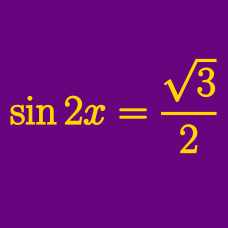Geometry

# Trigonometric Equations - Triple Angle Formula

In the domain $$[0, 2\pi]$$, how many solutions are there to

$\cos 3 \theta = 4 \cos \theta + 3 ?$

If provided that $\sin x+\cos x=\frac{1}{6},$ what is the value of $$\sin 3x-\cos 3x ?$$

Suppose that $$\displaystyle \cos \theta=\frac{\sqrt{2}}{4}$$ for $$\displaystyle 0 < \theta < \frac{\pi}{2}.$$ Then $$\sin 3\theta+\cos 3\theta$$ can be expressed as $\sin 3\theta+\cos 3\theta=\frac{a\sqrt{2}+b\sqrt{14}}{8}$ for rational numbers $$a$$ and $$b.$$ What is the value of $$3a+8b ?$$

If provided that $\sin x+\cos x=\frac{\sqrt{3}}{5},$ where $$\sin x > \cos x,$$ what is the value of $$\sin 3x+\cos 3x ?$$

Suppose that $$\sin 3\theta=\sin 2\theta$$ for $$\displaystyle 0 < \theta < \frac{\pi}{2}.$$ Then what is the value of $$20\cos^2 \theta-10\cos \theta ?$$

×

Problem Loading...

Note Loading...

Set Loading...